Скачать презентацию Chapter 8 Linear Regression Copyright 2009 Pearson

1a39e32c89887ae001584bb28ec0b74a.ppt

• Количество слайдов: 24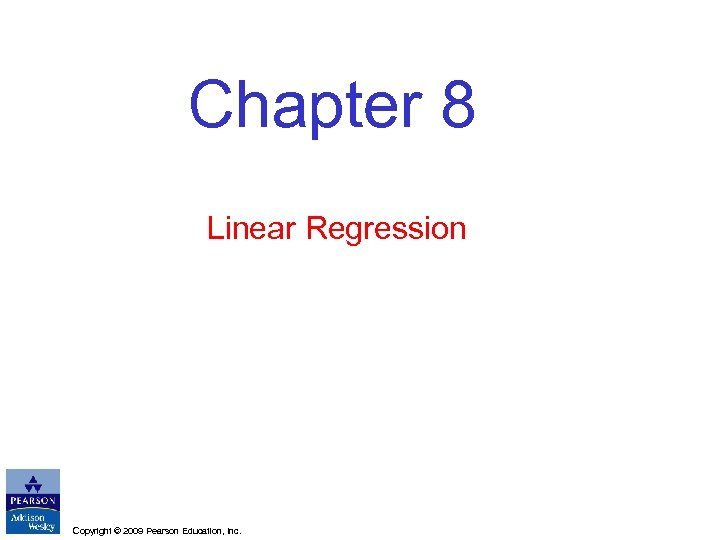Chapter 8 Linear Regression Copyright © 2009 Pearson Education, Inc.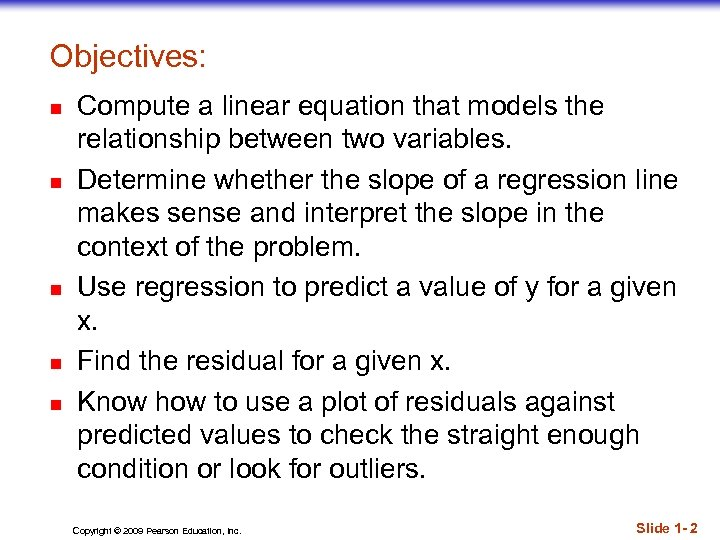Objectives: n n n Compute a linear equation that models the relationship between two variables. Determine whether the slope of a regression line makes sense and interpret the slope in the context of the problem. Use regression to predict a value of y for a given x. Find the residual for a given x. Know how to use a plot of residuals against predicted values to check the straight enough condition or look for outliers. Copyright © 2009 Pearson Education, Inc. Slide 1 - 2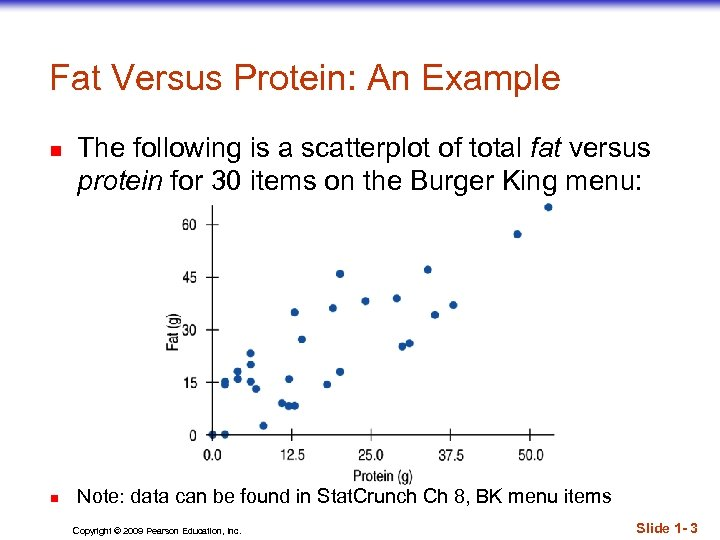Fat Versus Protein: An Example n n The following is a scatterplot of total fat versus protein for 30 items on the Burger King menu: Note: data can be found in Stat. Crunch Ch 8, BK menu items Copyright © 2009 Pearson Education, Inc. Slide 1 - 3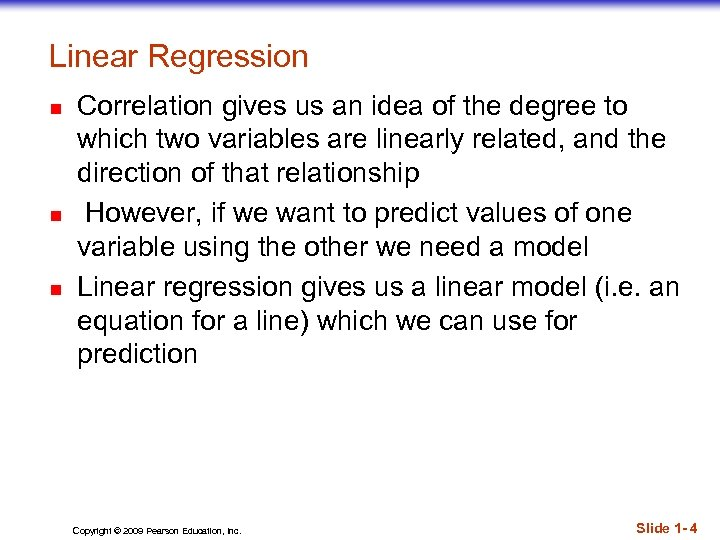Linear Regression n Correlation gives us an idea of the degree to which two variables are linearly related, and the direction of that relationship However, if we want to predict values of one variable using the other we need a model Linear regression gives us a linear model (i. e. an equation for a line) which we can use for prediction Copyright © 2009 Pearson Education, Inc. Slide 1 - 4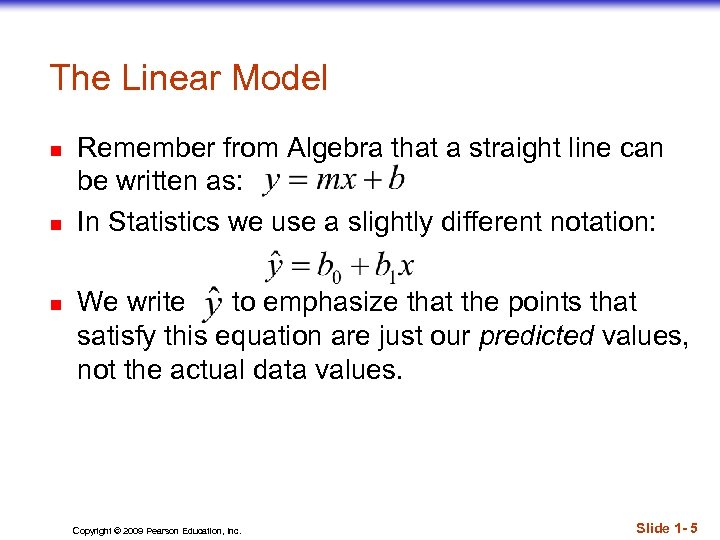The Linear Model n n n Remember from Algebra that a straight line can be written as: In Statistics we use a slightly different notation: We write to emphasize that the points that satisfy this equation are just our predicted values, not the actual data values. Copyright © 2009 Pearson Education, Inc. Slide 1 - 5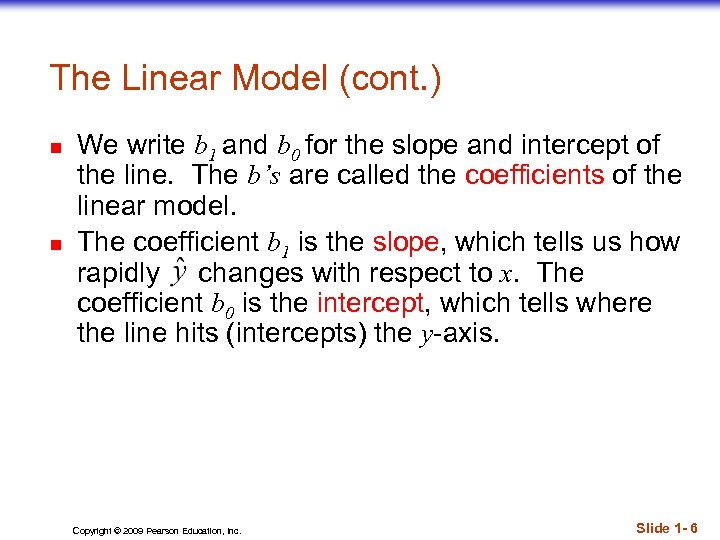The Linear Model (cont. ) n n We write b 1 and b 0 for the slope and intercept of the line. The b’s are called the coefficients of the linear model. The coefficient b 1 is the slope, which tells us how rapidly changes with respect to x. The coefficient b 0 is the intercept, which tells where the line hits (intercepts) the y-axis. Copyright © 2009 Pearson Education, Inc. Slide 1 - 6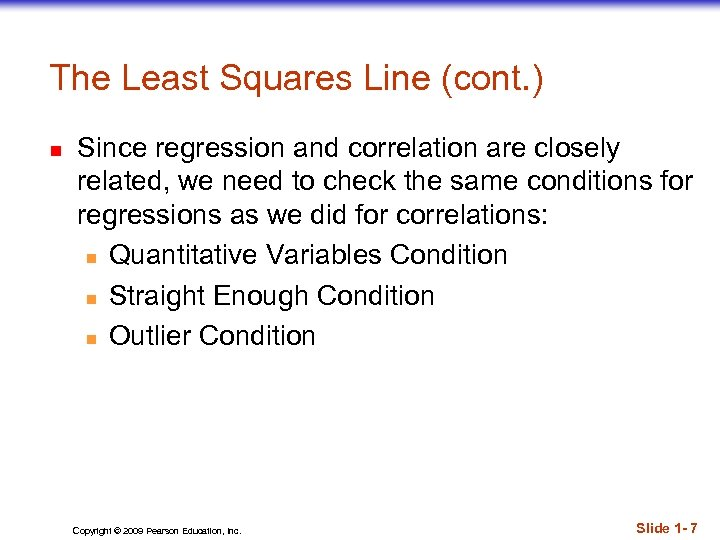The Least Squares Line (cont. ) n Since regression and correlation are closely related, we need to check the same conditions for regressions as we did for correlations: n Quantitative Variables Condition n Straight Enough Condition n Outlier Condition Copyright © 2009 Pearson Education, Inc. Slide 1 - 7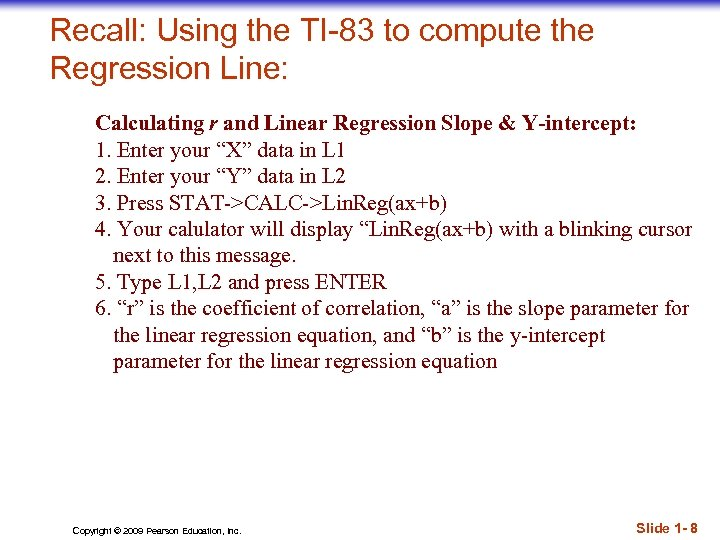Recall: Using the TI-83 to compute the Regression Line: Calculating r and Linear Regression Slope & Y-intercept: 1. Enter your “X” data in L 1 2. Enter your “Y” data in L 2 3. Press STAT->CALC->Lin. Reg(ax+b) 4. Your calulator will display “Lin. Reg(ax+b) with a blinking cursor next to this message. 5. Type L 1, L 2 and press ENTER 6. “r” is the coefficient of correlation, “a” is the slope parameter for the linear regression equation, and “b” is the y-intercept parameter for the linear regression equation Copyright © 2009 Pearson Education, Inc. Slide 1 - 8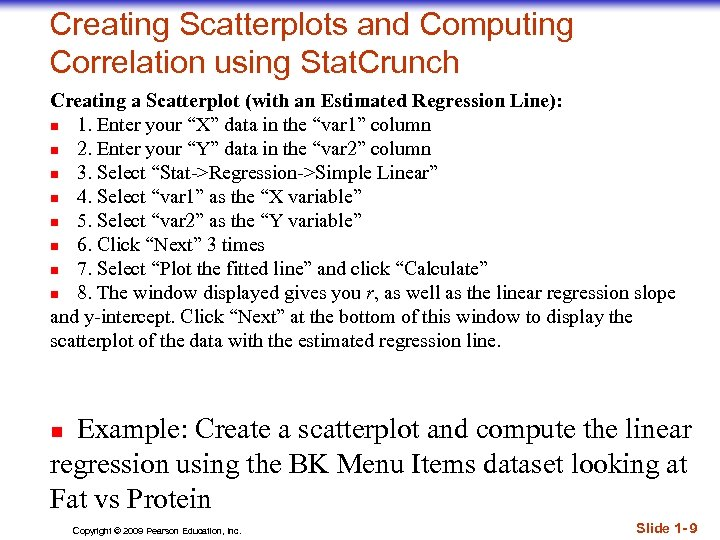Creating Scatterplots and Computing Correlation using Stat. Crunch Creating a Scatterplot (with an Estimated Regression Line): n 1. Enter your “X” data in the “var 1” column n 2. Enter your “Y” data in the “var 2” column n 3. Select “Stat->Regression->Simple Linear” n 4. Select “var 1” as the “X variable” n 5. Select “var 2” as the “Y variable” n 6. Click “Next” 3 times n 7. Select “Plot the fitted line” and click “Calculate” n 8. The window displayed gives you r, as well as the linear regression slope and y-intercept. Click “Next” at the bottom of this window to display the scatterplot of the data with the estimated regression line. Example: Create a scatterplot and compute the linear regression using the BK Menu Items dataset looking at Fat vs Protein n Copyright © 2009 Pearson Education, Inc. Slide 1 - 9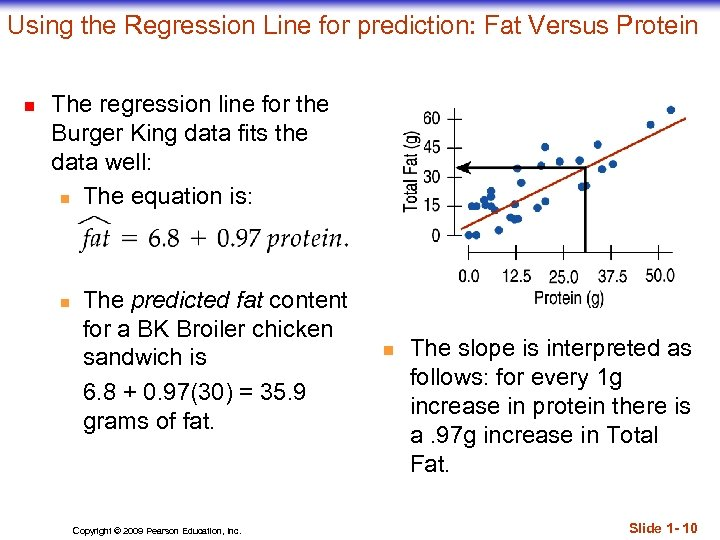Using the Regression Line for prediction: Fat Versus Protein n The regression line for the Burger King data fits the data well: n The equation is: n The predicted fat content for a BK Broiler chicken sandwich is 6. 8 + 0. 97(30) = 35. 9 grams of fat. Copyright © 2009 Pearson Education, Inc. n The slope is interpreted as follows: for every 1 g increase in protein there is a. 97 g increase in Total Fat. Slide 1 - 10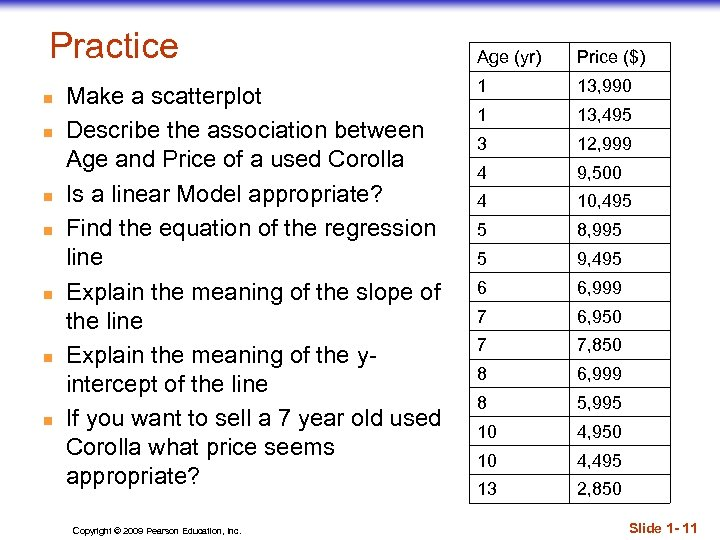Practice n n n n Make a scatterplot Describe the association between Age and Price of a used Corolla Is a linear Model appropriate? Find the equation of the regression line Explain the meaning of the slope of the line Explain the meaning of the yintercept of the line If you want to sell a 7 year old used Corolla what price seems appropriate? Copyright © 2009 Pearson Education, Inc. Age (yr) Price (\$) 1 13, 990 1 13, 495 3 12, 999 4 9, 500 4 10, 495 5 8, 995 5 9, 495 6 6, 999 7 6, 950 7 7, 850 8 6, 999 8 5, 995 10 4, 950 10 4, 495 13 2, 850 Slide 1 - 11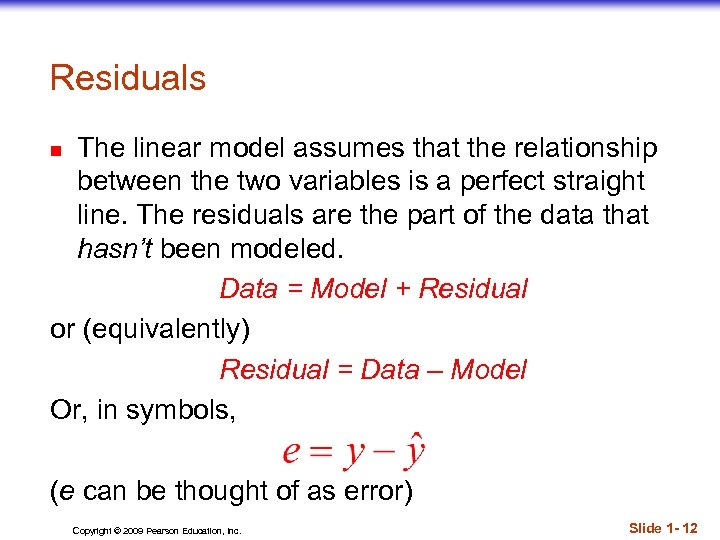Residuals The linear model assumes that the relationship between the two variables is a perfect straight line. The residuals are the part of the data that hasn’t been modeled. Data = Model + Residual or (equivalently) Residual = Data – Model Or, in symbols, n (e can be thought of as error) Copyright © 2009 Pearson Education, Inc. Slide 1 - 12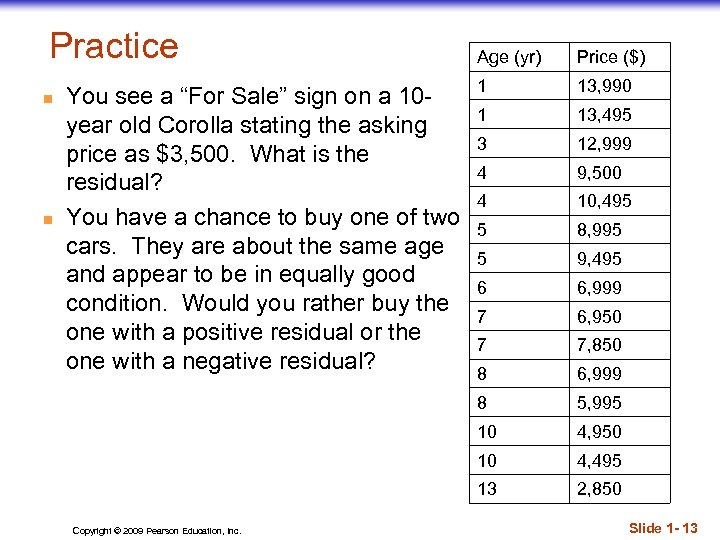Practice n n 1 13, 990 1 13, 495 3 12, 999 4 9, 500 4 10, 495 5 8, 995 5 9, 495 6 6, 999 7 6, 950 7 7, 850 8 6, 999 5, 995 10 4, 950 10 4, 495 13 Copyright © 2009 Pearson Education, Inc. Price (\$) 8 You see a “For Sale” sign on a 10 year old Corolla stating the asking price as \$3, 500. What is the residual? You have a chance to buy one of two cars. They are about the same age and appear to be in equally good condition. Would you rather buy the one with a positive residual or the one with a negative residual? Age (yr) 2, 850 Slide 1 - 13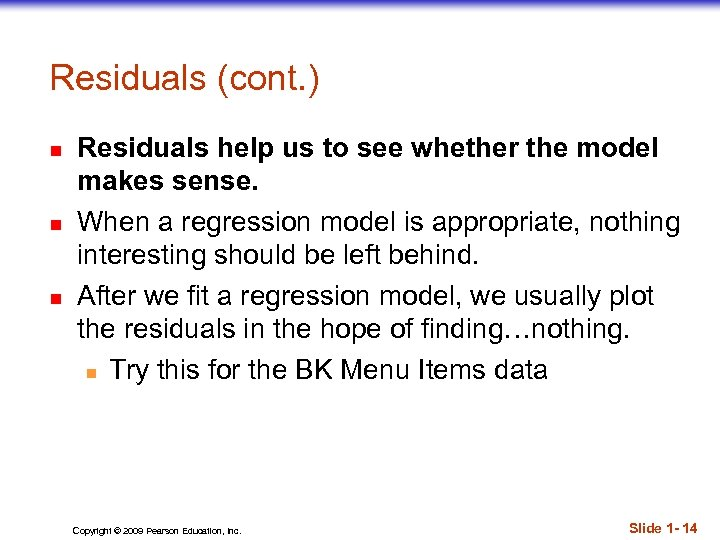Residuals (cont. ) n n n Residuals help us to see whether the model makes sense. When a regression model is appropriate, nothing interesting should be left behind. After we fit a regression model, we usually plot the residuals in the hope of finding…nothing. n Try this for the BK Menu Items data Copyright © 2009 Pearson Education, Inc. Slide 1 - 14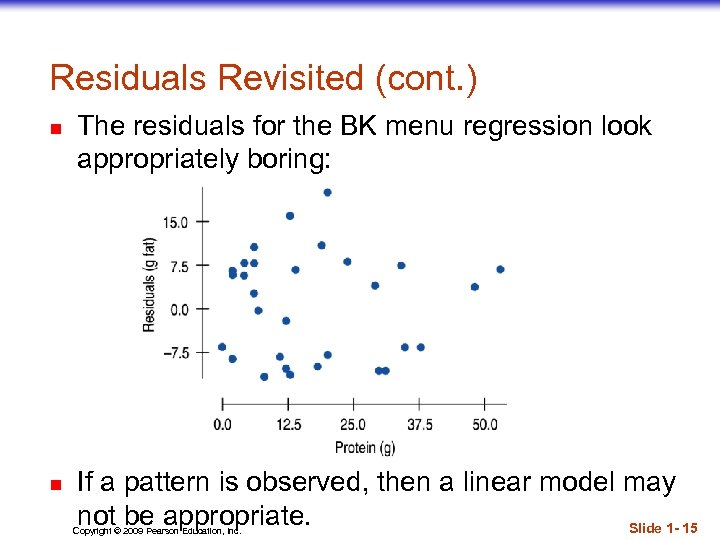Residuals Revisited (cont. ) n n The residuals for the BK menu regression look appropriately boring: If a pattern is observed, then a linear model may not be appropriate. Slide 1 - 15 Copyright © 2009 Pearson Education, Inc.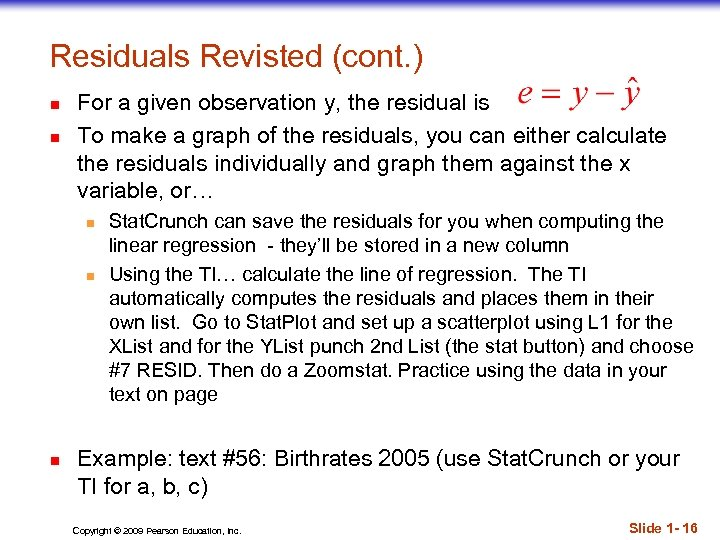Residuals Revisted (cont. ) n n For a given observation y, the residual is To make a graph of the residuals, you can either calculate the residuals individually and graph them against the x variable, or… n n n Stat. Crunch can save the residuals for you when computing the linear regression - they’ll be stored in a new column Using the TI… calculate the line of regression. The TI automatically computes the residuals and places them in their own list. Go to Stat. Plot and set up a scatterplot using L 1 for the XList and for the YList punch 2 nd List (the stat button) and choose #7 RESID. Then do a Zoomstat. Practice using the data in your text on page Example: text #56: Birthrates 2005 (use Stat. Crunch or your TI for a, b, c) Copyright © 2009 Pearson Education, Inc. Slide 1 - 16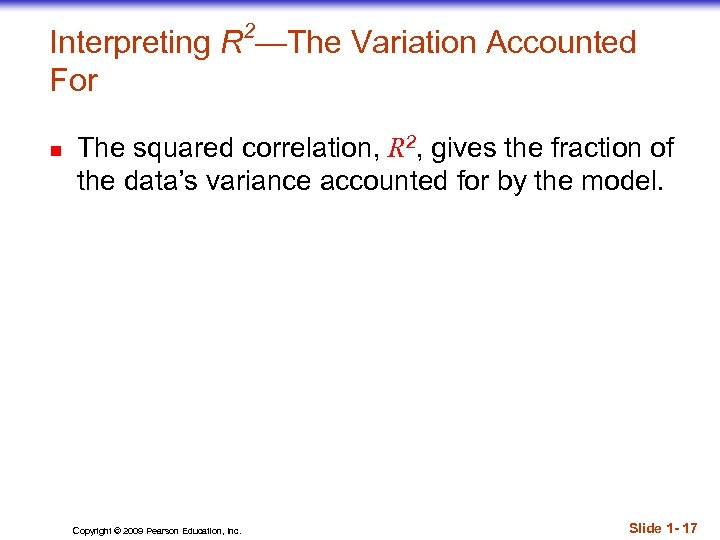2 Interpreting R —The Variation Accounted For n The squared correlation, R 2, gives the fraction of the data’s variance accounted for by the model. Copyright © 2009 Pearson Education, Inc. Slide 1 - 17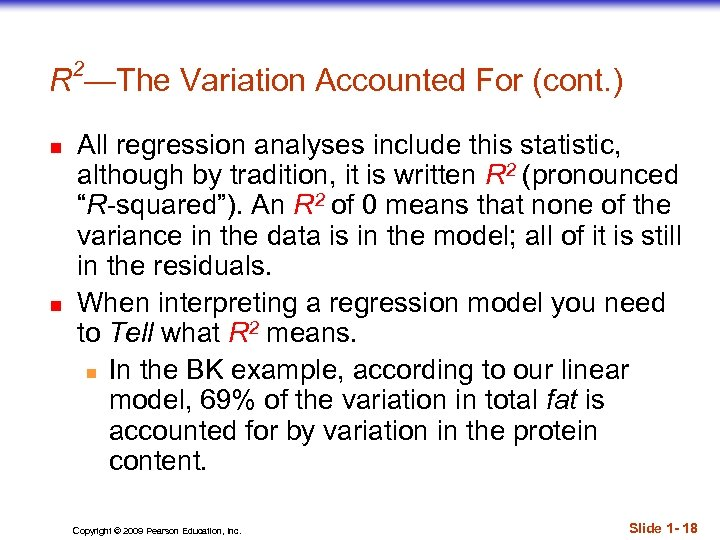R 2—The Variation Accounted For (cont. ) n n All regression analyses include this statistic, although by tradition, it is written R 2 (pronounced “R-squared”). An R 2 of 0 means that none of the variance in the data is in the model; all of it is still in the residuals. When interpreting a regression model you need to Tell what R 2 means. n In the BK example, according to our linear model, 69% of the variation in total fat is accounted for by variation in the protein content. Copyright © 2009 Pearson Education, Inc. Slide 1 - 18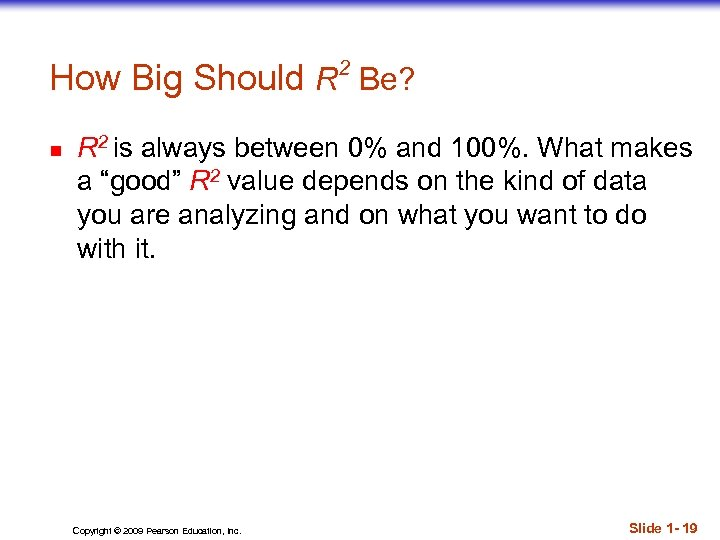2 How Big Should R Be? n R 2 is always between 0% and 100%. What makes a “good” R 2 value depends on the kind of data you are analyzing and on what you want to do with it. Copyright © 2009 Pearson Education, Inc. Slide 1 - 19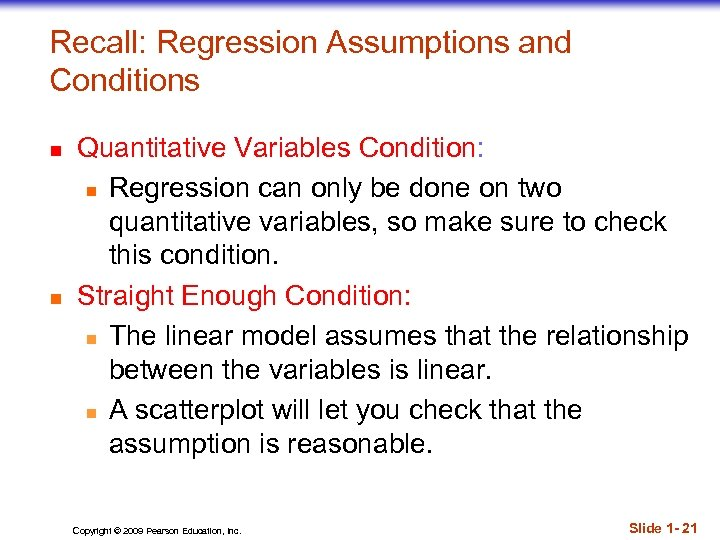Recall: Regression Assumptions and Conditions n n Quantitative Variables Condition: n Regression can only be done on two quantitative variables, so make sure to check this condition. Straight Enough Condition: n The linear model assumes that the relationship between the variables is linear. n A scatterplot will let you check that the assumption is reasonable. Copyright © 2009 Pearson Education, Inc. Slide 1 - 21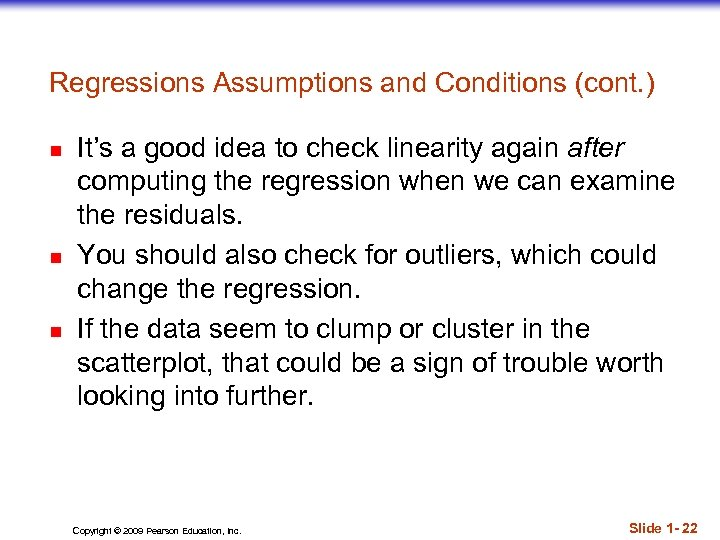Regressions Assumptions and Conditions (cont. ) n n n It’s a good idea to check linearity again after computing the regression when we can examine the residuals. You should also check for outliers, which could change the regression. If the data seem to clump or cluster in the scatterplot, that could be a sign of trouble worth looking into further. Copyright © 2009 Pearson Education, Inc. Slide 1 - 22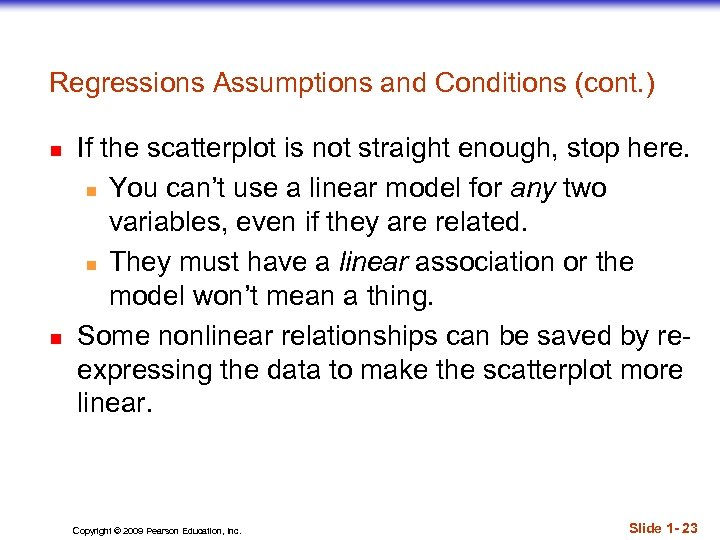Regressions Assumptions and Conditions (cont. ) n n If the scatterplot is not straight enough, stop here. n You can’t use a linear model for any two variables, even if they are related. n They must have a linear association or the model won’t mean a thing. Some nonlinear relationships can be saved by reexpressing the data to make the scatterplot more linear. Copyright © 2009 Pearson Education, Inc. Slide 1 - 23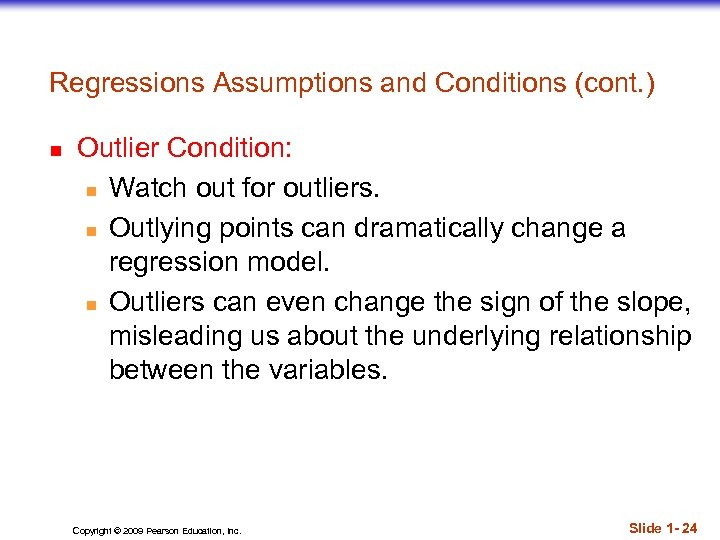Regressions Assumptions and Conditions (cont. ) n Outlier Condition: n Watch out for outliers. n Outlying points can dramatically change a regression model. n Outliers can even change the sign of the slope, misleading us about the underlying relationship between the variables. Copyright © 2009 Pearson Education, Inc. Slide 1 - 24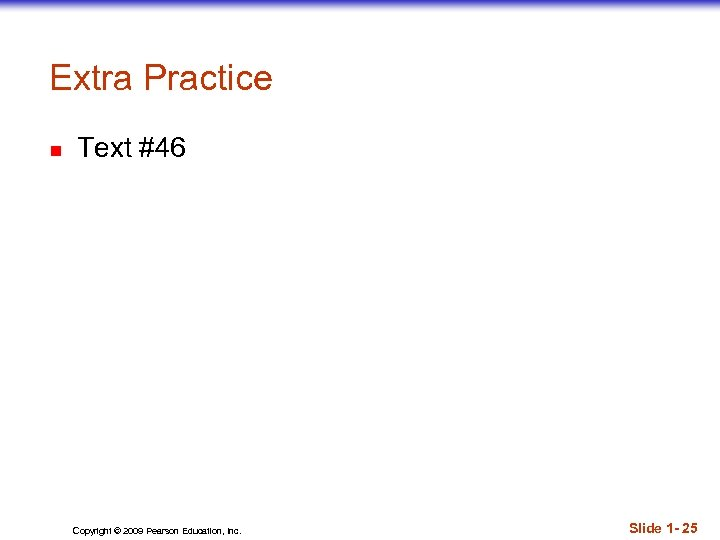Extra Practice n Text #46 Copyright © 2009 Pearson Education, Inc. Slide 1 - 25